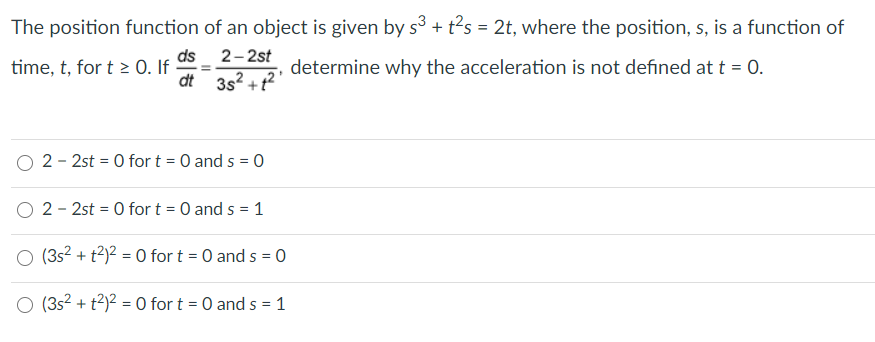# Question Solved1 AnswerThe position function of an object is given by s3 + t?s = 2t, where the position, s, is a function of 2-2st time, t, fort > 0. If determine why the acceleration is not defined at t = 0. dt 3s? +t?' ds O 2 - 2st = 0 for t = 0 and s = 0 O 2 - 2st = 0 for t = 0 and 5 = 1 (352 + 2)2 = 0 for t = 0 and 5 = 0 O (352 + 2)2 = 0 for t = 0 and 5 = 1Transcribed Image Text: The position function of an object is given by s3 + t?s = 2t, where the position, s, is a function of 2-2st time, t, fort > 0. If determine why the acceleration is not defined at t = 0. dt 3s? +t?' ds O 2 - 2st = 0 for t = 0 and s = 0 O 2 - 2st = 0 for t = 0 and 5 = 1 (352 + 2)2 = 0 for t = 0 and 5 = 0 O (352 + 2)2 = 0 for t = 0 and 5 = 1
More
Transcribed Image Text: The position function of an object is given by s3 + t?s = 2t, where the position, s, is a function of 2-2st time, t, fort > 0. If determine why the acceleration is not defined at t = 0. dt 3s? +t?' ds O 2 - 2st = 0 for t = 0 and s = 0 O 2 - 2st = 0 for t = 0 and 5 = 1 (352 + 2)2 = 0 for t = 0 and 5 = 0 O (352 + 2)2 = 0 for t = 0 and 5 = 1